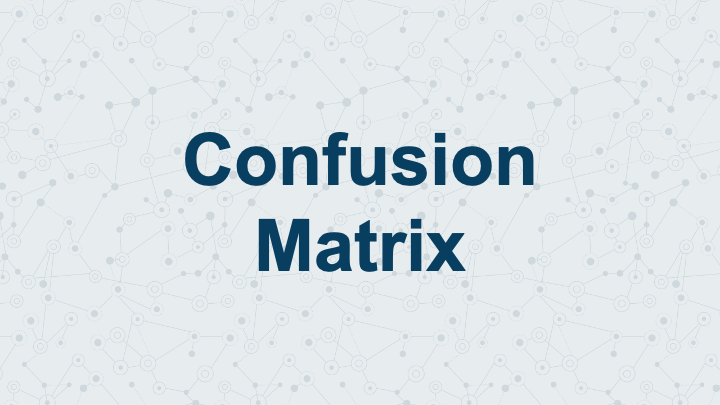Join thousands of AI enthusiasts and experts at the Learn AI Community.

## PublicationLatest

# Introduction to Confusion Matrix

Last Updated on January 7, 2023 by Editorial Team

Last Updated on September 23, 2022 by Editorial Team

#### Author(s): Saurabh Saxena

Originally published on Towards AI the World’s Leading AI and Technology News and Media Company. If you are building an AI-related product or service, we invite you to consider becoming an AI sponsor. At Towards AI, we help scale AI and technology startups. Let us help you unleash your technology to the masses.

#### What is Confusion Matrix, and how to plot it in Python?

The Confusion Matrix is the visual representation of the Actual VS Predicted values. It is a performance evaluation tool for classification algorithms, also known as the error matrix.

A two-dimensional table layout of how many predicted classes or categories were correctly predicted and how many were not allows visualization of the performance of an algorithm, typically in supervised learning.

In predictive analytics, a Confusion Matrix for binary classification is a table with two rows and two columns that reports the number of true positives, false negatives, false positives, and true negatives. This allows for more detailed analysis than simply observing accuracy.

### Why Confusion Matrix over Accuracy?

The accuracy metric can be misleading if used for the Imbalance dataset when the numbers of observations in different classes vary greatly. Whereas the Confusion Matrix provides a detailed comparison between Positives and Negatives.

Confusion Matrix consists of four important metrics True Positive(TP), True Negative(TN), False Positive(FP), False Negative(FN).

Let’s Understand them with an analogy where the algorithm has to categorize if a Person is Healthy or Sick.

(1) True Positive (TP)

The Algorithm predicted a “Person is Sick” who is Sick. This concludes that the algorithm has correctly classified the positive. It is the number of correct predictions when the actual class is positive.

(2) True Negative (TN)

The Algorithm predicted a “Person is Healthy” who is Healthy. This concludes that the algorithm has correctly classified the negative. It is the number of correct predictions when the actual class is negative.

(3) False Positive (FP)

The Algorithm predicted a “Person is Sick” who is Healthy. Here algorithm gave a false alarm by misclassifying it as Positive instead of Negative. It is the number of incorrect predictions when the actual class is positive, also referred to as Type I Error.

(4) False Negative (FN)

The Algorithm predicted a “Person is Healthy” who is Sick. Here algorithm missed a Sick Person by categorizing it healthy. It is the number of incorrect predictions when the actual class is negative, also referred to as Type II Error.

`from sklearn.datasets import load_breast_cancerfrom sklearn.model_selection import train_test_split from sklearn.linear_model import LogisticRegressionfrom sklearn.metrics import confusion_matrix`
`X, y = load_breast_cancer(return_X_y=True)X_train, X_test, y_train, y_test = train_test_split(X, y,                                                                                              test_size=0.33,                                                    random_state=42)lr= LogisticRegression() lr.fit(X_train,y_train) y_pred=lr.predict(X_test)confusion_matrix(y_test, y_pred)`
`Output:array([[ 63,   4],       [  3, 118]])`

The confusion_matrix API in sklearn provides an array as an output that has TN, FP, FN, and TP, respectively, and the same can be plotted using ConfusionMatrixDisplay API or Heatmap API of any visualization library.

Below is the python method for evaluating and plotting the Confusion matrix. It will give an array of tn, fp, fn, and tp as a return type and print the confusion matrix created by in seaborn theme.

`from sklearn.datasets import load_breast_cancerfrom sklearn.model_selection import train_test_split from sklearn.linear_model import LogisticRegressionfrom sklearn.metrics import confusion_matrix`
`X, y = load_breast_cancer(return_X_y=True)X_train, X_test, y_train, y_test = train_test_split(X, y,                                                                                              test_size=0.33,                                                    random_state=42)lr= LogisticRegression() lr.fit(X_train,y_train) y_pred=lr.predict(X_test)conf_mat, ax = confusion_matrix(y_test, y_pred)`

Below is the output for the code

The goal is to keep as many TP and TN values as possible.

In this blog, we understood what confusion Matrix is and How we can plot it in Python. Interpretation of True Positive(TP), True Negative(TN), False Positive(FP), and False Negative(FN) are the building metrics of the Confusion Matrix.

However, multiple metrics can be derived from the Confusion Matrix like Accuracy, Precision, Recall, ROC, and many more. Please refer to Deep dive into Confusion Matrix for details.

References:

 sklearn Confusion Matrix API. https://scikit-learn.org/stable/modules/generated/sklearn.metrics.confusion_matrix.html#sklearn.metrics.confusion_matrix

 sklearn ConfusionMatrixDisplay API. https://scikit-learn.org/stable/modules/generated/sklearn.metrics.ConfusionMatrixDisplay.html#sklearn.metrics.ConfusionMatrixDisplay

 seaborn Heatmap API. https://seaborn.pydata.org/generated/seaborn.heatmap.htmlIntroduction to Confusion Matrix was originally published in Towards AI on Medium, where people are continuing the conversation by highlighting and responding to this story.

Join thousands of data leaders on the AI newsletter. It’s free, we don’t spam, and we never share your email address. Keep up to date with the latest work in AI. From research to projects and ideas. If you are building an AI startup, an AI-related product, or a service, we invite you to consider becoming a sponsor.

Published via Towards AI